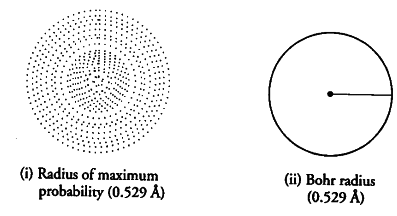# For H-atom the Bohr radius for first orbit is 0.529 A

For H-atom the Bohr radius for first orbit is 0.529 A and the radius of maximum probability for H-atom according to wave mechanical model is also 0.529 A. How do the two approaches differ?

Bohr found that the electron will always moving around the nucleus in a circular path of radius 0.529 A. He also suggested that electron cannot be found at distance less than or more than 0.529 A. However wave mechanical model suggest that electron is most likely to be found at this distance but there is definite probability of finding the electron at the distances shorter and larger than 0.529 A.In other words, according to wave mechanical model the electron keeps on moving towards or away from the nucleus and the maximum probability oPocating it is at radius of 0.529 A from the nucleus. Figure (i) indicates the electron cloud picture of lr orbital having radius of maximum probability and Figure (ii) indicates the Bohr picture of orbit in which electron is found only at this distance.